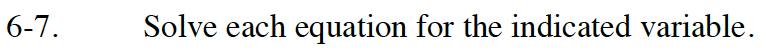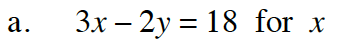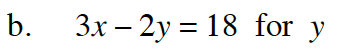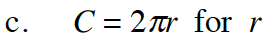Home > GB8I > Chapter cc46 > Lesson cc46.1.1 > Problem6-7

6-7.
1. Solve each equation for the indicated variable. Homework Help ✎

1. 3x − 2y = 18 for x

2. 3x − 2y = 18 for y

3. C = 2πr for rAdd 2y to both sides.
3x = 18 + 2y

Divide both sides by 3.

$x=6+\frac{2}{3}\textit{y}$See part (a).

$y=\frac{3}{2}x-9$Divide both sides by 2π.